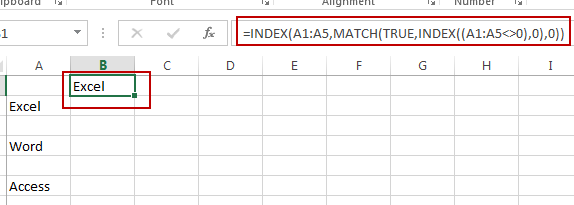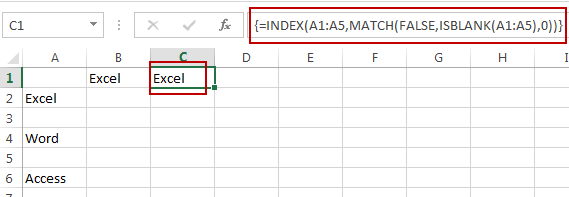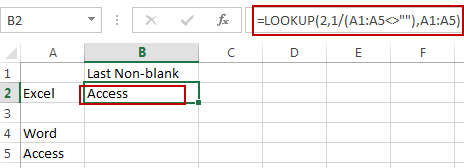# How to Find the First or Last Non-blank Cell in a Row or Column in Excel

This post will guide you how to find the first non-blank cell in a row or column or a range in Excel. How do I get the value of the last non-blank cell in a row or column using formula in Excel.

## Get First Non-blank Cell Value

Assuming that you have a list of data in range A1:A5, and you want to find the first non-blank cell in a given range of cells in Excel. How to do it. You can use a formula based on the INDEX function and the MATCH function to get the first non-blank value in a one-column range in Excel. Here is the formula you can use:

`=INDEX(A1:A5,MATCH(TRUE,INDEX((A1:A5<>0),0),0))`

Type this formula into a blank cell and press Enter key on your keyboard. You would get the first non-blank cell value from your given range A1:A5.Or you can use an array formula based on the INDEX function, the MATCH function and the ISBLANK function to achieve the same result of extracting the first non-blank cell value from a given range in Excel. Like this:

`=INDEX(A1:A5,MATCH(FALSE,ISBLANK(A1:A5),0))`

Type this formula into a blank cell and press Ctrl + Shift + Enter keys on your keyboard to make your formula as array formula.## Get Last Non-blank Cell Value

If you want to find the value of the last non-blank cell in a row or column in Excel, you can use a formula based on the LOOKUP function to achieve it. Here is the formula we are using is:

`=LOOKUP(2,1/(A1:A5<>""),A1:A5)`

Type this formula into a blank cell and press Enter key on your keyboard. You would get the last non-blank cell value from your given range A1:A5.### Related Functions

• Excel LOOKUP function
The Excel LOOKUP function will search a value in a vector or array.The syntax of the LOOKUP function is as below:= LOOKUP (lookup_value, lookup_vector, [result_vector])…
• Excel INDEX function
The Excel INDEX function returns a value from a table based on the index (row number and column number)The INDEX function is a build-in function in Microsoft Excel and it is categorized as a Lookup and Reference Function.The syntax of the INDEX function is as below:= INDEX (array, row_num,[column_num])…
• Excel MATCH  function
The Excel MATCH function search a value in an array and returns the position of that item.The MATCH function is a build-in function in Microsoft Excel and it is categorized as a Lookup and Reference Function.The syntax of the MATCH function is as below:= MATCH  (lookup_value, lookup_array, [match_type])….
• Excel ISBLANK function
The Excel ISBLANK function returns TRUE if the value is blank or null.The syntax of the ISBLANK function is as below:= ISBLANK (value)…

Related Posts

Break ties with helper COUNTIF and column

Suppose you got a task to adjust the values that contain the ties; what would be your first attempt to break the ties of the given value? If you are wondering about doing this task manually, let me add that ...

Find the Closest Data to the Data Provided in Excel

In our daily work, we may encounter such an issue that to find the closest value to a certain value. In fact, Excel internal functions can help us solve this problem. In today’s article, we will show you how to ...

Abbreviate Names Or Words in Excel

As an MS Excel user, you might have come across a task where you need to abbreviate different names or words, and there are also possibilities that you might have done this task manually by assuming that there isn't any ...

Convert State Names To Abbreviations

Assume that you got a task to convert the full state's name into the abbreviations in MS Excel, and for doing this task, you might choose to do it manually, which is an acceptable way only if you don't have ...

Filter or Remove Columns

Assume that in MS Excel, you have a table consisting of a few columns consisting of few values, and you want to filter to remove the specified columns from the table. You might take it easy and would prefer to ...

Filter or Extract for Multiple OR Criteria

If you are an valid MS Excel user, you have probably come across a situation where you wanted to filter the data in a separate table with specific criteria. You could do this task manually, which is also acceptable when ...

Filter Multiple Values

In Excel, you can easily filter a table to display only the rows that meet your criteria. This is a quick way to find the information you need without scrolling through all the data. In this post, we'll show you ...

Excel XLOOKUP Function

Excel XLOOKUP Function was added into Excel as a beta feature in August 2019 and is now accessible exclusively in Microsoft 365. (as of July 2021). However, if you fall into this category and often deal with big sets of ...

Extract or Filter Top n values

You might have been through this kind of situation where you need to filter out the top n values from the list having few values, and I am also pretty sure about it that you might have chosen to do ...

Extract Unique Items From A List

This post will guide you how to extract unique itmes from a given list in Microsoft Excel. How to create a newly formula to get unique values from a range cells in Excel. The unique list of items is the ...

Sidebar